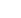# 4.1.2 Elimination of Left-Recursion

There is a formal technique for eliminating left-recursion from productions

Step One: Direct-Recursion

For each rule which contains a left-recursive option,

A --> A|introduce a new nonterminal A' and rewrite the rule as

A -->A'
A' -->|A'

Thus the production:

E --> E + T | T

is left-recursive with "E" playing the role of "A","+ T" playing the role of, and "T" playing the role ofA'. Introducing the new nonterminal E', the production can be replaced by:

E --> T E'
E' -->| + T E'

Of course, there may be more than one left-recursive part on the right-hand side. The general rule is to replace:

A --> A|| ...||| ... |by

A -->A' |A'| ...|A'
A' -->|A' |A' | ...|A'

Note that this may change the "structure". For the expression above, the original grammar is left-associative, while the non-left recursive one is now right-associative.

Step Two: Indirect-Recursion

Step one describes a rule to eliminate direct left recursion from a production. To eliminate left-recursion from an entire grammar may be more difficult because of indirect left-recursion. For example,

``` ```

``` A --> B x y | x B --> C D C --> A | c D --> d ```
``` ``` is indirectly recursive because

``` A ==> B x y ==> C D x y ==> A D x y. ```

``` ```

That is, ` A ==> ... ==> A` whereis ` D x y. `

The algorithm eliminates left-recursion entirely. It contains a "call" to a procedure which eliminates direct left-recursion (as described in step one).

Example 3 illustrates this algorithm for the grammar of Example 3.Send questions and comment to: Karen Lemone